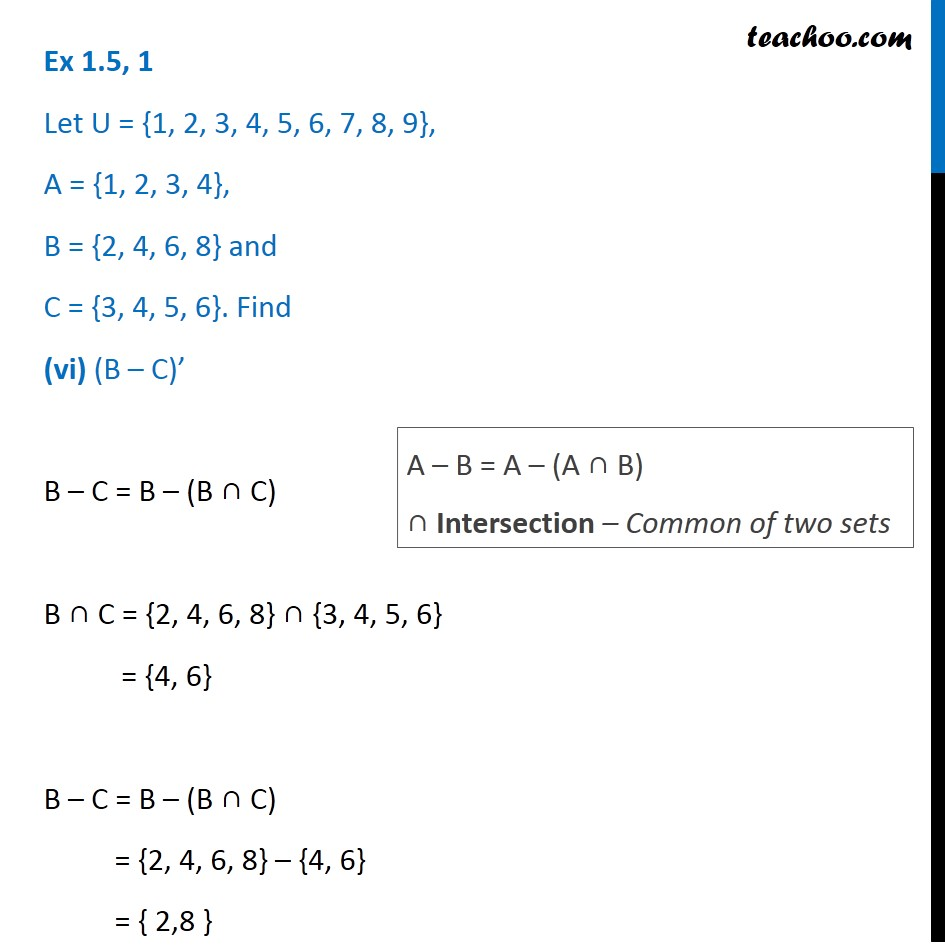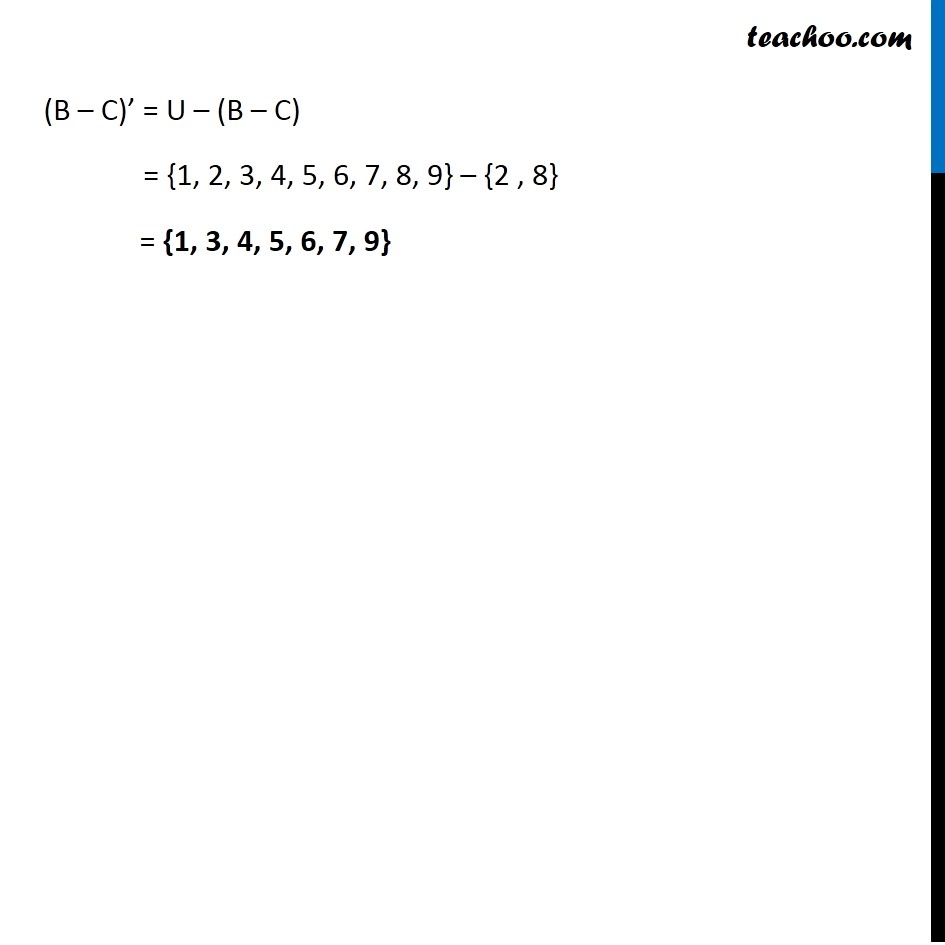1. Chapter 1 Class 11 Sets (Term 1)
2. Serial order wise
3. Ex 1.5

Transcript

Ex 1.5, 1 Let U = {1, 2, 3, 4, 5, 6, 7, 8, 9}, A = {1, 2, 3, 4}, B = {2, 4, 6, 8} and C = {3, 4, 5, 6}. Find (vi) (B – C)’ B – C = B – (B ∩ C) B ∩ C = {2, 4, 6, 8} ∩ {3, 4, 5, 6} = {4, 6} B – C = B – (B ∩ C) = {2, 4, 6, 8} – {4, 6} = { 2,8 } A – B = A – (A ∩ B) ∩ Intersection – Common of two sets (B – C)’ = U – (B – C) = {1, 2, 3, 4, 5, 6, 7, 8, 9} – {2 , 8} = {1, 3, 4, 5, 6, 7, 9}